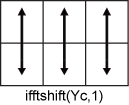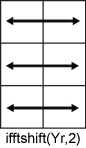# ifftshift

## 语法

``X = ifftshift(Y)``
``X = ifftshift(Y,dim)``

## 说明

``X = ifftshift(Y)` 将进行过零频平移的傅里叶变换 `Y` 重新排列回原始变换输出的样子。换言之，`ifftshift` 就是撤消 `fftshift` 的结果。如果 `Y` 是向量，则 `ifftshift` 会将 `Y` 的左右两半部分进行交换。如果 `Y` 是矩阵，则 `ifftshift` 会将 `Y` 的第一象限与第三象限交换，将第二象限与第四象限交换。如果 `Y` 是多维数组，则 `ifftshift` 会沿每个维度交换 `Y` 的半空间。`

``X = ifftshift(Y,dim)` 沿 `Y` 的维度 `dim` 执行运算。例如，如果 `Y` 是矩阵，其行表示多个一维变换，则 `ifftshift(Y,2)` 会将 `Y` 的每一行的左右两半部分进行交换。`

## 示例

```V = [1 2 3 4 5 6 7]; X = fftshift(V)```
```X = 1×7 5 6 7 1 2 3 4 ```

`Y = ifftshift(X)`
```Y = 1×7 1 2 3 4 5 6 7 ```

`fftshift(fftshift(V))`
```ans = 1×7 2 3 4 5 6 7 1 ```

```Y = [-2 -1 0 1 2; -10 -5 0 5 10]; X = ifftshift(Y,2)```
```X = 2×5 0 1 2 -2 -1 0 5 10 -10 -5 ```

## 输入参数

• 以输入矩阵 `Yc` 为例。运算 `ifftshift(Yc,1)` 会将 `Yc` 的每一列的上下两半部分进行交换。• 以输入矩阵 `Yr` 为例。运算 `ifftshift(Yr,2)` 会将 `Yr` 的每一行的左右两半部分进行交换。